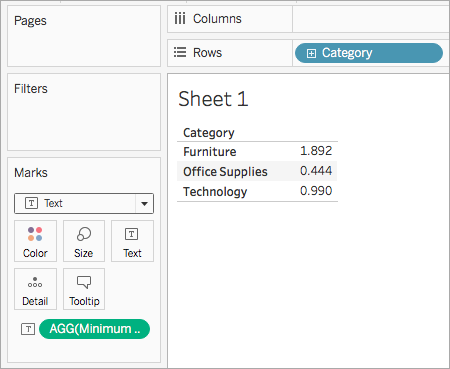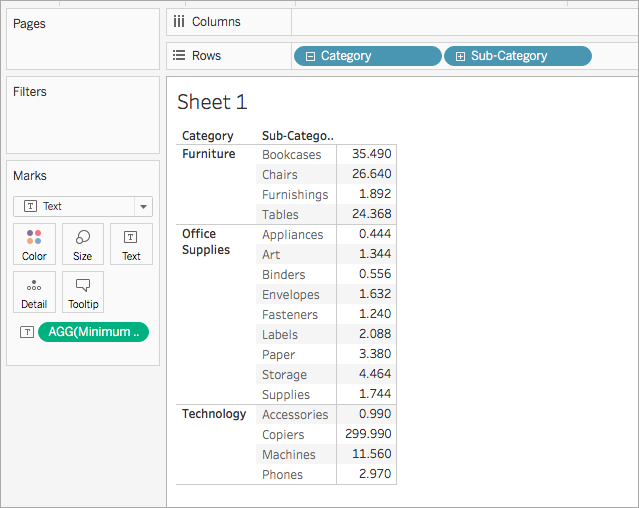# Number Functions

This article introduces number functions and their uses in Tableau. It also demonstrates how to create a number calculation using an example.

## Why use number functions

Number functions allow you to perform computations on the data values in your fields. Number functions can only be used with fields that contain numerical values. For more information, see Data Types.

For example, you might have a field that contains values for the variance in your budget, titled Budget Variance. One of those values might be -7. You can use the ABS function to return the absolute value of that number, and all the other numbers in that field.

The calculation might look something like this:

`ABS[Budget Variance]`

And for that -7 value, the output would be 7.

## Number functions available in Tableau

### ABS

 Syntax `ABS(number)` Output Number (positive) Definition Returns the absolute value of the given ``. Example `ABS(-7) = 7ABS([Budget Variance])` The second example returns the absolute value for all the numbers contained in the Budget Variance field. Notes See also `SIGN`.

### ACOS

 Syntax `ACOS(number)` Output Number (angle in radians) Definition Returns the arccosine (angle) of the given ``. Example `ACOS(-1) = 3.14159265358979` Notes The inverse function, `COS`, takes the angle in radians as the argument and returns the cosine.

### ASIN

 Syntax `ASIN(number)` Output Number (angle in radians) Definition Returns the arcsine (angle) of a given ``. Example `ASIN(1) = 1.5707963267949` Notes The inverse function, `SIN`, takes the angle in radians as the argument and returns the sine.

### ATAN

 Syntax `ATAN(number)` Output Number (angle in radians) Definition Returns the arctangent (angle) of a given ``. Example `ATAN(180) = 1.5652408283942` Notes The inverse function, `TAN`, takes the angle in radians as the argument and returns the tangent. See also `ATAN2` and `COT`.

### ATAN2

 Syntax `ATAN2(y number, x number)` Output Number (angle in radians) Definition Returns the arctangent (angle) between two numbers (x and y). The result is in radians. Example `ATAN2(2, 1) = 1.10714871779409` Notes See also `ATAN`, `TAN`, and `COT`.

### CEILING

 Syntax `CEILING(number)` Output Integer Definition Rounds a `` to the nearest integer of equal or greater value. Example `CEILING(2.1) = 3` Notes See also `FLOOR` and `ROUND`. Database limitations `CEILING` is available through the following connectors: Microsoft Excel, Text File, Statistical File, Published Data Source, Amazon EMR Hadoop Hive, Amazon Redshift, Cloudera Hadoop, DataStax Enterprise, Google Analytics, Google BigQuery, Hortonworks Hadoop Hive, MapR Hadoop Hive, Microsoft SQL Server, Salesforce, Spark SQL.

### COS

 Syntax `COS(number)` The number argument is the angle in radians. Output Number Definition Returns the cosine of an angle. Example `COS(PI( ) /4) = 0.707106781186548` Notes The inverse function, `ACOS`, takes the cosine as the argument and returns the angle in radians. See also `PI`. To convert an angle from degrees to radians, use `RADIANS`.

### COT

 Syntax `COT(number)` The number argument is the angle in radians. Output Number Definition Returns the cotangent of an angle. Example `COT(PI( ) /4) = 1` Notes See also `ATAN`, `TAN`, and `PI`. To convert an angle from degrees to radians, use `RADIANS`.

### DEGREES

 Syntax `DEGREES(number)` The number argument is the angle in radians. Output Number (degrees) Definition Converts an angle in radians to degrees. Example `DEGREES(PI( )/4) = 45.0` Notes The inverse function, `RADIANS`, takes an angle in degrees and returns the angle in radians. See also `PI()`.

### DIV

 Syntax `DIV(integer1, integer2)` Output Integer Definition Returns the integer part of a division operation, in which `` is divided by ``. Example `DIV(11,2) = 5`

### EXP

 Syntax `EXP(number)` Output Number Definition Returns e raised to the power of the given ``. Example `EXP(2) = 7.389EXP(-[Growth Rate]*[Time])` Notes See also `LN`.

### FLOOR

 Syntax `FLOOR(number)` Output Integer Definition Rounds a number to the nearest `` of equal or lesser value. Example `FLOOR(7.9) = 7` Notes See also `CEILING` and `ROUND`. Database limitations `FLOOR` is available through the following connectors: Microsoft Excel, Text File, Statistical File, Published Data Source, Amazon EMR Hadoop Hive, Cloudera Hadoop, DataStax Enterprise, Google Analytics, Google BigQuery, Hortonworks Hadoop Hive, MapR Hadoop Hive, Microsoft SQL Server, Salesforce, Spark SQL.

### HEXBINX

 Syntax `HEXBINX(number, number)` Output Number Definition Maps an x, y coordinate to the x-coordinate of the nearest hexagonal bin. The bins have side length 1, so the inputs may need to be scaled appropriately. Example `HEXBINX([Longitude]*2.5, [Latitude]*2.5)` Notes `HEXBINX `and `HEXBINY` are binning and plotting functions for hexagonal bins. Hexagonal bins are an efficient and elegant option for visualizing data in an x/y plane such as a map. Because the bins are hexagonal, each bin closely approximates a circle and minimizes variation in the distance from the data point to the center of the bin. This makes the clustering both more accurate and informative.

### HEXBINY

 Syntax `HEXBINY(number, number)` Output Number Definition Maps an x, y coordinate to the y-coordinate of the nearest hexagonal bin. The bins have side length 1, so the inputs may need to be scaled appropriately. Example `HEXBINY([Longitude]*2.5, [Latitude]*2.5)` Notes See also `HEXBINX`.

### LN

 Syntax `LN(number)` Output Number The output is `Null` if the argument is less than or equal to zero. Definition Returns the natural logarithm of a ``. Example `LN(50) = 3.912023005` Notes See also `EXP` and `LOG`.

### LOG

 Syntax `LOG(number, [base])` If the optional base argument isn't present, base 10 is used. Output Number Definition Returns the logarithm of a number for the given base. Example `LOG(16,4) = 2` Notes See also `POWER` `LN`.

### MAX

 Syntax `MAX(expression)` or `MAX(expr1, expr2)` Output Same data type as the argument, or `NULL `if any part of the argument is null. Definition Returns the maximum of the two arguments, which must be of the same data type. `MAX` can also be applied to a single field as an aggregation. Example `MAX(4,7) = 7MAX(#3/25/1986#, #2/20/2021#) = #2/20/2021# MAX([Name]) = "Zander"` Notes For strings `MAX` is usually the value that comes last in alphabetical order. For database data sources, the `MAX` string value is highest in the sort sequence defined by the database for that column. For dates For dates, the `MAX` is the most recent date. If `MAX` is an aggregation, the result will not have a date hierarchy. If `MAX` is a comparison, the result will retain the date hierarchy. As an aggregation `MAX(expression)` is an aggregate function and returns a single aggregated result. This displays as `AGG(expression)` in the viz. As a comparison `MAX(expr1, expr2)` compares the two values and returns a row-level value. See also `MIN`.

### MIN

 Syntax `MIN(expression)` or `MIN(expr1, expr2)` Output Same data type as the argument, or `NULL `if any part of the argument is null. Definition Returns the maximum of the two arguments, which must be of the same data type. `MIN `can also be applied to a single field as an aggregation. Example `MIN(4,7) = 4MIN(#3/25/1986#, #2/20/2021#) = #3/25/1986#MIN([Name]) = "Abebi"` Notes For strings `MIN` is usually the value that comes first in alphabetical order. For database data sources, the `MIN` string value is lowest in the sort sequence defined by the database for that column. For dates For dates, the `MIN` is the earliest date. If `MIN` is an aggregation, the result will not have a date hierarchy. If `MIN` is a comparison, the result will retain the date hierarchy. As an aggregation `MIN(expression)` is an aggregate function and returns a single aggregated result. This displays as `AGG(expression)` in the viz. As a comparison `MIN(expr1, expr2)` compares the two values and returns a row-level value. See also `MAX`.

### PI

 Syntax `PI()` Output Number Definition Returns the numeric constant pi: 3.14159... Example `PI() = 3.14159` Notes Useful for trig functions that take their input in radians. See also `RADIANS`.

### POWER

 Syntax `POWER(number, power)` Output Number Definition Raises the `` to the specified ``. Example `POWER(5,3) = 125POWER([Temperature], 2)` Notes You can also use the ^ symbol, such as `5^3 = POWER(5,3) = 125`See also `EXP`, `LOG`, and `SQUARE`.

 Syntax `RADIANS(number)` Output Number (angle in radians) Definition Converts the given `` from degrees to radians. Example `RADIANS(180) = 3.14159` Notes The inverse function, `DEGREES`, takes an angle in radians and returns the angle in degrees.

### ROUND

 Syntax `ROUND(number, [decimals])` Output Number Definition Rounds `` to a specified number of digits. The optional `decimals` argument specifies how many decimal points of precision to include in the final result. If `decimals` is omitted, number is rounded to the nearest integer. Example `ROUND(1/3, 2) = 0.33` Notes Some databases, such as SQL Server, allow specification of a negative length, where -1 rounds number to 10's, -2 rounds to 100's, and so on. This is not true of all databases. For example, it is not true of Excel or Access. Tip: Because `ROUND` may run into issues due to the underlying floating point representation of numbers—such as 9.405 rounding to 9.40—it may be preferable to format the number to the desired number of decimal points rather than rounding. Formatting 9.405 to two decimal places will yield the expected 9.41. See also `CEILING` and `FLOOR`.

### SIGN

 Syntax `SIGN(number)` Output -1, 0, or 1 Definition Returns the sign of a ``: The possible return values are -1 if the number is negative, 0 if the number is zero, or 1 if the number is positive. Example `SIGN(AVG(Profit)) = -1` Notes See also `ABS`.

### SIN

 Syntax `SIN(number)` The number argument is the angle in radians. Output Number Definition Returns the sine of an angle. Example `SIN(0) = 1.0SIN(PI( )/4) = 0.707106781186548` Notes The inverse function, `ASIN`, takes the sine as the argument and returns the angle in radians. See also `PI`. To convert an angle from degrees to radians, use `RADIANS`.

### SQRT

 Syntax `SQRT(number)` Output Number Definition Returns the square root of a ``. Example `SQRT(25) = 5` Notes See also `SQUARE`.

### SQUARE

 Syntax `SQUARE(number)` Output Number Definition Returns the square of a ``. Example `SQUARE(5) = 25` Notes See also `SQRT` and `POWER`.

### TAN

 Syntax `TAN(number)` The number argument is the angle in radians. Output Number Definition Returns the tangent of an angle. Example `TAN(PI ( )/4) = 1.0` Notes See also `ATAN`, `ATAN2`,`COT`, and `PI`. To convert an angle from degrees to radians, use `RADIANS`.

### ZN

 Syntax `ZN(expression)` Output Any, or o Definition Returns the `` if it is not null, otherwise returns zero. Use this function to replace null values with zeros. Example `ZN(Grade) = 0` Notes This is a very useful function when using fields that may contain nulls in a calculation. Wrapping the field with `ZN` can prevent errors caused by calculating with nulls.

## Create a number calculation

Follow along with the steps below to learn how to create a number calculation.

1. In Tableau Desktop, connect to the Sample - Superstore saved data source, which comes with Tableau.

2. Navigate to a worksheet and select Analysis > Create Calculated Field.

3. In the calculation editor that opens, do the following:

• Name the calculated field Minimum Sales transaction

• Enter the following formula:

`MIN(Sales)`

• When finished, click OK.

The new number calculation appears under Measures in the Data pane. Just like your other fields, you can use it in one or more visualizations.

When Minimum Sales is placed on Text on the Marks card in the worksheet, its name is changed to AGG(Minimum Sales), which indicates that it cannot be aggregated any further, since it is already aggregated down to the lowest level of detail (the smallest sales value for all records).

This example shows the minimum sales per category.When subcategory is brought into the view, the minimum sales for each subcategory are shown.